Definitions of Square Dance Calls and Concepts
Quick Step | {anything} [C3A]

Index -->  Plus  |  A1  |  A2  |  C1  |  C2  |  C3A  |  C3B  |  C4  |  NOL  |
Definitions (Text Only) -->  Plus  |  A1  |  A2  |  C1  |  C2  |  C3A  |  C3B  |  C4  |  NOL  |
 Find call:

 \$B8@8l(BQuick Step [C3A]: 1/4 Box \$B\$^\$?\$OB>\$NE,@Z\$J(B formation \$B\$+\$i!%(B2 \$BHVL\\$N?M\$O(B Press Out \$B\$r\$7\$F(B, \$BNY\$N?M\$H(B Phantom Ah So \$B\$r\$7(B, \$B\$=\$NB>\$N?M\$O(B Circulate \$B\$r\$7\$^\$9!%(B1/4 Box \$B\$+\$i\$O(B Column \$B\$G=*\$o\$j\$^\$9!%(B

Quick anything [C3A]: 1/4 Box \$B\$^\$?\$O(B Column \$B\$+\$i!%(B2 \$BHVL\\$N?M\$O(B Press Out \$B\$r\$7\$F(B, \$B2q\$C\$??M\$H(B (\$BI,MW\$J\$i(B Phantom \$B\$H(B) anything \$B\$N%3!<%k\$r\$7(B, \$B\$=\$NB>\$N?M\$O(B Circulate \$B\$r\$7\$^\$9!%(B Outsides \$B\$H(B centers \$B\$N4V\$K(B Phantom \$B\$,\$G\$-\$k\$H\$-\$O(B, \$B%3!<%k\$N=*\$o\$j\$G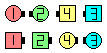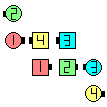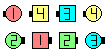Quick Step\$B\$NA0(B #2 Press Out \$BNY\$j\$KC/\$bMh\$J\$+\$C\$??M\$,(B Circulate \$B\$N8e(B Outsides Ah So\$B\$N8e(B (\$B=*\$o\$j(B)

\$BCm

• Quick Step \$B\$O(B Quick Ah So \$B\$G\$9!%(B
• anything \$B\$N%3!<%k\$,(B 'Cycle' \$B\$@\$C\$?\$i(B Recycle \$B\$r\$7\$^\$9!%(B
•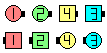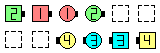Quick Cross Fire\$B\$NA0(B \$B8e(B

Magic Quick Step [C3A]: 2 \$BHVL\\$N?M\$O(B Magic Press Out (\$B30B&\$N%+%C%W%k\$NN>NY\$j\$N\$&\$A(B, \$B9T\$-\$K\$/\$\$J}\$X(B) \$B\$r\$7(B, \$B\$=\$N?M\$H(B Phantom Ah So \$B\$r\$7\$F(B, \$B\$=\$NB>\$N?M\$O(B Magic Column Circulate \$B\$r\$7\$^\$9!%(B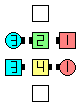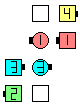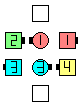Center 6 Magic Quick Step\$B\$NA0(B #2 Magic Press Out\$BNY\$j\$KC/\$bMh\$J\$+\$C\$??M\$,(B Magic Circulate \$B\$N8e(B Outsides Ah So\$B\$N8e(B (\$B=*\$o\$j(B)

\$B1~MQ(B: Magic Quick anything.CALLERLAB definition for Quick StepCALLERLAB definition for Quick AnythingChoreography for Quick Step | anythingComments? Questions? Suggestions?

https://www.ceder.net/def/quick.php?level=master&language=japan
06-December-2019 03:46:57